# Can anyone help me answer the question

## Recommended Posts

The establishment of the Maxwell equations marks the perfection of the classical physics. But it is resolved from the Maxwell equations that the velocity of light is a constant. To explain the confict between the Maxwell equations and the classical physics, Lorentz revised the Galilean transformation to Lorentz transformation. Since the invariableness of the light velocity is veried by Michelson and Morley experiment, the light velocity has been accepted by people as a constant, and thus the special relativity put forward by Einstein.
According to the special relativity theory equation: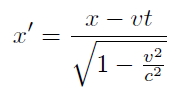Suppose that R1 and R2 are both inertial reference systems whose relative velocity is u. If an object is static relative to R1 and the length of the object is L0 in the X axis direction. And in reference system R2, the measured length of the object is L. Then the relationship between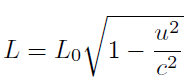The following experiment is designed in the inertial reference system R1: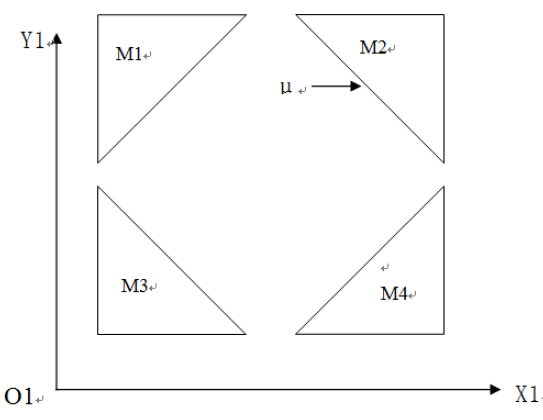in above picture, M1, M2, M3 and M4 are four total reflection lens, whose cross-sectional shape are isosceles right triangles. Photonμis eradiate into the surface of M2 with  the incidence angle of 45 degree. It will be reflected among the four lens. Assume energy is conservative during the reflection process, the photon μ will always stay in the cage of the four lens and vibrate forever.

Now there is another inertial reference system R2, Which is moving at a constant speed in direction of X axis compared to R1. If observed in R2, according to the second equation the four lens of M1, M2, M3 and M4 shrink in the direction X and remain stable in direction Y as illustrated in the following picture: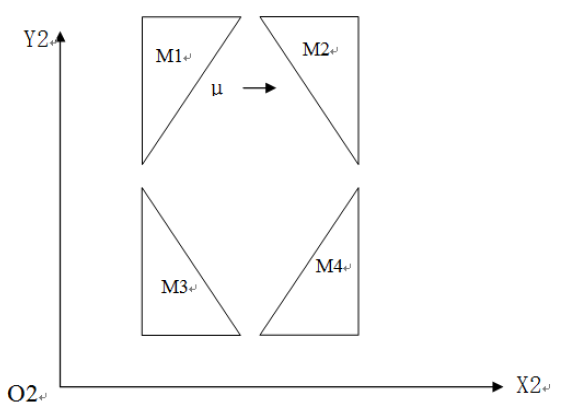Because the lens are deformed in R2, the Photon μ will not in parallel with Y after being reflected by M2. Thus the photon μ will escape from the gap between M1, M2, M3 and M4 after several times of reflection.

So in the two reference systems above, two different results will be observed for one thing, which is in conflict with the fact that everything has its uniqueness.

The essence of the Maxwell’s equations and the permanent principle of light velocity

The Maxwell equations are as follows: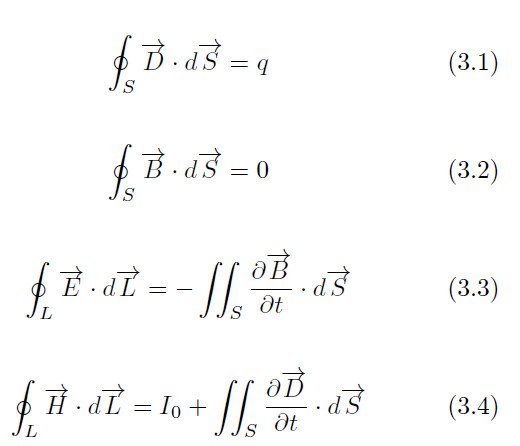Among which, the experimental basis for equation 3.1 is the coulomb’s law. The experimental basis for equation 3.2 is the Biot-Savart’s Law.  The equation 3.3 is deduced from  Faraday’s law of electromagnetic induction and the equation 3.4 is deduced from the Ampere circuital theorem and Maxwell’s hypothesis.

During the solving process of the equations, Maxwell did not mention the reference system. But the Coulomb’s law and the Ampere circuital theorem are the conclusions based on the experiment in the reference system of the earth. So the Maxwell’s equations cannot be regarded as have nothing to do with the reference system. It is not scientific to discuss the Maxwell’s equations apart from the reference system.

Now a new theory will be brought to explain the constancy of the speed of light and the experimental result of Michelson-Morley.

The experiments done by great scientists such as Coulomb, Ampere, and Faraday are two objects that remain stationary. We define this as the reference frame 1. If we observe at reference frame 1 in another relative motion reference frame 2, the experimental reference frame 1 has a certain velocity, but the two objects in the experiment remain relatively stationary, so I have reason to conclude:

The speed of the electromagnetic wave is constant relative to its emission source.

Electromagnetic wave comes from the emission source. The Maxwell’s equations did not mention the reference system. The experimental speed of the electromagnetic is in such accordance with the solution of the Maxwell’s equations. So it can be derived that the speed of the electromagnetic wave is constant relative to the emission source. The scientists are always relatively static to the emission source when testing the velocity of the electromagnetic wave thus the testing result are always and must be a fixed value.

From this theory we can deduce that the experiment data and conclusion of Coulomb, Ampere and Faraday are all correct. Because in their experiment the electric charge, the magnet, the conductor and the coil are static relative to the reference system of the earth. So the speed of electromagnetic wave is constant. The positon of the static charge and its electric field is fixed. The positon of the magnet and it magnetic field is also fixed. But if the observer is moving relative to the experimental reference system of the emit source, the tested velocity of the electromagnetic wave will not be a constant, which comply with the speed superposition principle. Thus the Doppler effect of Electromagnetic wave can be observed.

Now let’s go back to the Morley and Michelson’s experiment. To explain the result of the experiment, we need to rely on theory one. The relative speed of the reflected light to the reflector is constant. So in the Morley and Michelson’s experiment, the emission light source, stripe interference plate and the translucent glass in the middle are relatively static. That is to say,The geometric space formed by the objects in the experiment is a constant value with respect to the wave lengthλof the light. Thus no matter how the experiment system turns or no matter whether it moves with the earth, the shift of the interference figure will not be observed.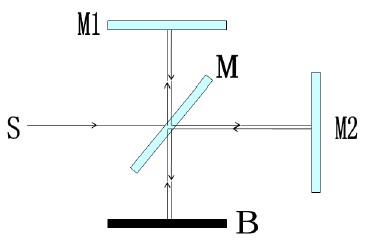In the above figure besides the light source S, the pellicle mirror M and the reflecting mirror M1/M2 can also be regard as light source. So the speed of the light reflected or emitted by them are constant C (velocity of light).

The most important experiment to support the theory of relativity is that a charged particle can never be accelerated to the speed of light by a linear accelerator or cyclotron. The higher the speed of the charged particle is, the more difficult for it to be accelerated. Thus the theory of relativity is put forward: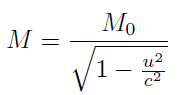According to the Hubble’s law, the universe is expanding and the farther the distance between the stars and the earth, the bigger the radial regression rate. Suppose there are two stars in the faraway place which are moving away from the earth with the velocity of light. The two stars are near to each other. Then seen from the earth the mass of the two stars are infinite in the process of high-speed regression. The gravity of them will be infinite too .Ultimately the two stars will merge together. Otherwise seen from the double star reference system which are separated with the light velocity, the earth and the sun will merge together by the appealing force of the infinite mass while it is definitely not true.

The essence of force is interaction. But without the interaction, we can never perceive the existence of the objects let alone to measure the force. In the view of the conventional concept, the interaction between objects is unchangeable. Now a new theory is put forward: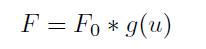The interaction force between objects is changing with the relative radial velocity of the object.

g(u) is the transformation factor , which is the radial velocity between objects. The following transformation equation on F and F0 can be estimated based on equation below.(only I guessed)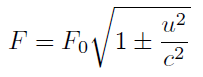In the above formula, F0 is the apply force with the relative speed of the accelerating field is zero. “+”stand for the backward motion to the accelerate field. “-”stand for the forward motion to the accelerate field.

Now we know that the particle can’t be accelerated beyond velocity c by an electric field accelerator in the earth. While in another reference system the velocity stack still exist which is to say in different reference system the moving speed of an object can exceed c. that is why it is not necessary to rectify the Galileo transformation to Lorentz transformation or add a reference system for the Maxwell’s equations.

Note that in the Theory of Relativity force is also a covariate. But the deduction is based on the change of the mass. The transformation formula of force is the derivation result of the momentum equation. In the Theory of Relativity many variables are covariate. While in this theory the mass, the time and the length are constant value.

Edited by ruibin.niu
Need to delete a redundant picture
##### Share on other sites

1 hour ago, ruibin.niu said:

But the Coulomb’s law and the Ampere circuital theorem are the conclusions based on the experiment in the reference system of the earth. So the Maxwell’s equations cannot be regarded as have nothing to do with the reference system. It is not scientific to discuss the Maxwell’s equations apart from the reference system.

It is trivially easy to write the Maxwell equations in covariant form, i.e. without reference to any particular coordinate system:

$dF=0$

$d \star F=\mu_0 \star J$

These are valid for any reference system, both in free space, and in curved spacetimes. The version you gave is one written in terms of 3-vectors, which directly follows from the above when using a Cartesian coordinate system.

##### Share on other sites

We consider the first equation of Maxwell's equation, which is obtained by the closed surface integration of Coulomb's law. Coulomb's law does not consider whether the earth is an inertial reference frame. The law of gravitation does not consider whether the earth is gravitational or not. The inertial reference system, Coulomb constant K and gravitational constant K can all be accurately measured on the earth. If the mass and distance in the theory of relativity vary with the reference system, the constants tested in different reference systems should be different. However, in the solar system, the gravitational constant is fixed, and we rely on it to launch artificial spacecraft.

##### Share on other sites

26 minutes ago, ruibin.niu said:

Coulomb's law does not consider whether the earth is an inertial reference frame.

It doesn't need to, because it is equally valid in all reference frames, whether inertial or not. Also, the earth is of course never 'in an inertial frame'.
Nonetheless, these laws are sufficiently well tested in purely inertial frames as well, e.g. in satellites and the ISS. Evidently, Maxwell's laws work just as well there.

26 minutes ago, ruibin.niu said:

The law of gravitation does not consider whether the earth is gravitational or not.

The law of gravitation is the field equations of GR, and as a tensorial equation they are also equally valid in all reference frames.

26 minutes ago, ruibin.niu said:

If the mass and distance in the theory of relativity vary with the reference system, the constants tested in different reference systems should be different.

The gravitational field equations are a system of differential equations, so they are a purely local constraint on local geometry - hence there are of course no changes in any constants.

26 minutes ago, ruibin.niu said:

However, in the solar system, the gravitational constant is fixed, and we rely on it to launch artificial spacecraft

Exactly. The calculation of probe trajectories is done using GR, and it evidently yields very accurate and precise results. This shows just how accurate the theory of relativity is.

Edited by Markus Hanke
##### Share on other sites

5 hours ago, ruibin.niu said:

We consider the first equation of Maxwell's equation, which is obtained by the closed surface integration of Coulomb's law. Coulomb's law does not consider whether the earth is an inertial reference frame. The law of gravitation does not consider whether the earth is gravitational or not. The inertial reference system, Coulomb constant K and gravitational constant K can all be accurately measured on the earth. If the mass and distance in the theory of relativity vary with the reference system, the constants tested in different reference systems should be different. However, in the solar system, the gravitational constant is fixed, and we rely on it to launch artificial spacecraft.

What errors does this introduce, and how big are they? You can solve for the effects when you accelerate an electron, for instance.

11 hours ago, ruibin.niu said:

Now there is another inertial reference system R2, Which is moving at a constant speed in direction of X axis compared to R1. If observed in R2, according to the second equation the four lens of M1, M2, M3 and M4 shrink in the direction X and remain stable in direction Y as illustrated in the following picture:

Because the lens are deformed in R2, the Photon μ will not in parallel with Y after being reflected by M2. Thus the photon μ will escape from the gap between M1, M2, M3 and M4 after several times of reflection.

You say this, but have not actually shown it to be true. You have not done any analysis of the light path in the moving frame when accounting for the motion of the light.

edit to add: consider light traveling vertically and hitting a small aperture. If that frame is moving to the right, the light also has a component of motion to the right — it still hits the aperture. (this has empirical support)

Your assertion that the light would escape appears to fail to take this into account.

##### Share on other sites

!

Moderator Note

Moved to Speculations as it seems the OP is not just confused, but promoting a novel theory.

##### Share on other sites

7 hours ago, swansont said:

You say this, but have not actually shown it to be true. You have not done any analysis of the light path in the moving frame when accounting for the motion of the light.

Very good point. +1

While it is true that you've show an intuition of something unexpected, we know how all kinds of very similar naive intuitions fail one by one when you apply the formalism.

Can you provide equations, showing the velocities and the angles in both inertial frames, or are you expecting everybody else to believe it only based on intuition? Do you want somebody else to do the analysis for you?

IOW: Why should anyone bother with this?

##### Share on other sites

9 hours ago, swansont said:

edit to add: consider light traveling vertically and hitting a small aperture. If that frame is moving to the right, the light also has a component of motion to the right — it still hits the aperture. (this has empirical support)

I did a bit of "common sense" analysis, just enough to resolve any apparent paradox in my mind.

The path of a photon in the moving R2 frame would look something like this:

___   ___   ___   ->
\_/   \_/   \_/

The angled legs are symmetric, and would have a length (or light time) of gamma times their length in the stationary frame.

Due to aberration of light, the different mirrors would appear skewed in the R2 frame, in different ways depending on where the observer is. Do you happen to know, if there was a stationary mirror in the R2 frame, angled so that it matched the appearance of a moving skewed mirror, would light take the same path if it hit that stationary mirror as it would hitting the moving mirror? It seems it would but I'm not sure (a problem with using only common sense). That would mean that the light always appears to reflect at the "correct" angle with nothing visually paradoxical.

Edit: It seems it wouldn't. Differently positioned observers sharing the R2 frame would see a moving mirror appearing skewed differently, but see the path of light being the same. The angle of reflection would change somewhat like a ping pong ball hit by a moving paddle.

5 hours ago, Strange said:
!

Moderator Note

Moved to Speculations as it seems the OP is not just confused, but promoting a novel theory.

Is it now off-topic to discuss the relativity-related aspects of the topic? We should focus on the speculation? Should we start a new topic in Relativity if we don't want the thread killed when OP says the wrong thing, or is discussion of relativity just generally discouraged? (And would a new thread be killed if new OP says the wrong thing, eventually leading to the ideal condition where only people who already understand relativity can start a topic, but none start topics, and the Relativity forum is perfectly devoid of any active topics?)

Edited by md65536
##### Share on other sites

1 hour ago, md65536 said:

Is it now off-topic to discuss the relativity-related aspects of the topic?

!

Moderator Note

I don't think that would be off topic, as the thread appears to be about relativity (and the OP's misunderstandings).

##### Share on other sites

2 hours ago, md65536 said:

Edit: It seems it wouldn't. Differently positioned observers sharing the R2 frame would see a moving mirror appearing skewed differently, but see the path of light being the same. The angle of reflection would change somewhat like a ping pong ball hit by a moving paddle.

Oh, you're sooo right. +1. I will rephrase the flaw in the OP's argument: Snell's law, as is, does not hold for moving mirrors.

Edited by joigus
##### Share on other sites

On 7/27/2020 at 6:05 AM, Markus Hanke said:

Drawing a picture takes time. Draw a picture to explain how photons escape from the system.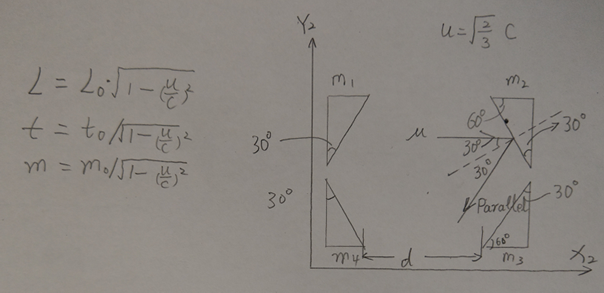As shown in the figure, the second frame of reference R2 moves to the right at a speed of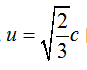. It can be seen from formula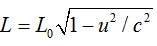that the side parallel to the X axis of the original isosceles right triangle total reflection lens is shortened to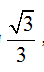, so the angle is also reduced from 45° to 30° ,as the picture shows.
Therefore, after the photon u is incident parallel to the X axis, it is emitted in a symmetrical direction along the normal line, parallel to the reflecting surface of the mirror m3, that is to say, the photon u will not enter m3 after being reflected by m2 for the first time, and the size of d does not affect The total reflection of the reference frame R1, but if the distance d is large enough, then the first reflection of the photon will pass through the gap between m3 and m4.
The ping pang model of Mr md65536 is that photons are reflected between two parallel surfaces, which is different from the experiment I constructed.

Edited by Strange
Fixed quote
##### Share on other sites

The quote in the previous post by ruibin.niu isn’t actually from me - I don’t know how my name got on that. This just for the record.

##### Share on other sites

37 minutes ago, Markus Hanke said:

The quote in the previous post by ruibin.niu isn’t actually from me - I don’t know how my name got on that. This just for the record.

Yeah, I don't know what you did with the quoting function there, @ruibin.niu. Be careful.

6 hours ago, ruibin.niu said:

Drawing a picture takes time. Draw a picture to explain how photons escape from the system.

I think there are some merits to your gedanken, but you got the analysis wrong. Careful application of SR formulas (Einstein's velocity transformation law) gives you that,

$\theta_{i}<\theta_{r}$

While you're assuming that Snell's law,

$\theta_{i}=\theta_{r}$

is correct.

It is not for moving mirrors. You can find the derivation in Landau-Lifshitz; Classical Field Theory, Vol. II. You have a larger reflection angle than incident angle, which accounts for the relative direction of light rays in the $v_{\parallel}$ for both observers to coincide and the whole picture to be consistent.

##### Share on other sites

1 hour ago, Markus Hanke said:

The quote in the previous post by ruibin.niu isn’t actually from me - I don’t know how my name got on that. This just for the record.

Fixed. (Another data point for my spreadsheet(*) on the correlation between "personal theory" and "unable to use quote function".)

(*) Purely imaginary, unfortunately.

##### Share on other sites

14 hours ago, ruibin.niu said:

Therefore, after the photon u is incident parallel to the X axis, it is emitted in a symmetrical direction along the normal line, parallel to the reflecting surface of the mirror m3, that is to say, the photon u will not enter m3 after being reflected by m2 for the first time, and the size of d does not affect The total reflection of the reference frame R1, but if the distance d is large enough, then the first reflection of the photon will pass through the gap between m3 and m4.
The ping pang model of Mr md65536 is that photons are reflected between two parallel surfaces, which is different from the experiment I constructed.

Actually, I think you can prove to yourself that a moving mirror must be able to change the angle of reflection. Try this: Have a box with a mirror on one interior surface, and two holes such that if you shone a beam of light through one, you could reflect it off the mirror and out the other hole. Consider that the stationary frame. Now in a moving frame (in a direction of the mirror's normal), the box is moving while the light makes its way from one hole, to the mirror, and out the other hole. If you draw this on paper using 3 positions for the box for when light enters, reflects, and exits, you'll see that the angle must be different than in the stationary frame. You can try this with or without length contraction (you'd have other contradictions), the angle of reflection is not going to be the same in all frames.

##### Share on other sites

Thank you all for your guidance.
A friend said to consider the Lorentz transformation, but the earth is not an inertial frame of reference. The reflection experiment on the earth proved that the angle of incidence is equal to the angle of reflection, and the law of reflection needs to be consistent in all frames of reference.
The following is my calculation process.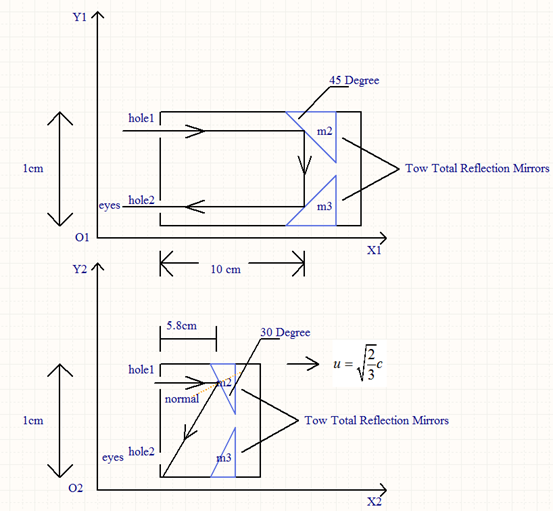The first picture is the reference frame 1. There are two total reflection mirrors m2, m3 inside the box. After a beam of light enters through hole 1, since the reflector is 45 degrees, it will be emitted from hole 2 after reflection by m2 and m3 and enter Human eyes.

Now the box moves to the right at a speed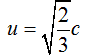of  relative to the reference frame 2. The original isosceles right-angled triangle total reflection mirror, the side parallel to the X axis is shortened, and the angle is reduced from 45 degrees to 30 degrees, as shown in the second figure.

Therefore, after the light enters parallel to the X axis, it exits in the symmetrical direction of the normal, parallel to the reflecting surface of the mirror m3, that is to say, the photon will not enter m3 after being reflected by m2 for the first time, and the box can be on the X axis The direction design is very long, and the design in the Y-axis direction is very short. Even if the box moves at a high speed to the right, the light will reach the bottom edge of the box first and will not be emitted from hole 2. The following is the calculation process:

The time required for the beam reflected from m2 to reach the bottom edge of the box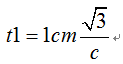In time t1, the distance the box moves is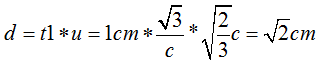,this is less than 5.8cm, so the light beam reaches the bottom edge of the box first, and will not be emitted from hole 2.

If the box is long enough, the beam cannot be emitted from hole 2.

##### Share on other sites

On 7/29/2020 at 6:15 AM, ruibin.niu said:

Thank you all for your guidance.

I'm not aware that you've accepted any guidance here. Please, correct me if I'm wrong. You've just pressed your initial point while ignoring all the counter-arguments.

On 7/29/2020 at 6:15 AM, ruibin.niu said:

[...] and the law of reflection needs to be consistent in all frames of reference.

Can you show that? I'm not convinced. "It needs to be consistent" is obviously not good enough. I don't think that's what the Einstein transformation of velocities implies.

##### Share on other sites

For the observer to whom the apparatus is length contracted, the mirrors are moving. I figure that can't be ignored as light has a finite speed.

##### Share on other sites

On 7/29/2020 at 5:15 AM, ruibin.niu said:

and the law of reflection needs to be consistent in all frames of reference

No, the law of reflection does not hold at relativistic velocities.

##### Share on other sites

9 hours ago, pzkpfw said:

For the observer to whom the apparatus is length contracted, the mirrors are moving. I figure that can't be ignored as light has a finite speed.

Yes, I mentioned this earlier.

In the basic "light clock" explanation, the light is incident normal to the mirror in the vertical direction in the rest frame, but that angle is not the same when you do the analysis in the moving frame — the light has a component of motion in the x-direction, for which one must account. It's the same concept here, only now you do not have normal incidence.

##### Share on other sites

• 3 weeks later...

## Create an account

Register a new account